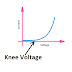## What is Knee Voltage? Definition

The knee voltage is known for the voltage applied to semiconductor devices such as PN junction Diodes, Silicon controlled rectifiers, etc. For a PN junction diode, the minimum applied positive voltage at which the junction rapidly expands and the PN junction Diode starts to conduct current through it is called knee voltage.

We know that when the positive terminal of the power source is connected to the cathode of the PN Junction Diode and the negative terminal of the power source is connected to the anode terminal of the PN Junction Diode, it is called Reverse Bias and in this condition, the Diode does not conduct any current even we increase the applied voltage.

But when the positive terminal of the power source is connected to the anode terminal of the PN Junction Diode and the negative terminal of the power source is connected to the cathode terminal of the diode, it is called forward bias. In this condition, the applied voltage has a minimum value that must be greater than the barrier potential of the diode. Once the applied voltage exceeds the barrier potential the diode starts to conduct current. And this minimum voltage above the barrier potential is responsible for conducting current is known as Knee voltage.

The knee voltage is also known as cut-in voltage. After the knee voltage, the conducting current will increase proportionally to the increase in the applied voltage.

### Knee Voltage Formula

Actually, there is no particular formula to find the accurate knee voltage of a PN junction device but the Current Transformer or CT has a formula or equation for knee voltage. That is,

Vkp = K * If/CTR x (RCT + RL + RR)

Here,

K = Constant, usually taken as 2.0

Vkp = The least Knee Point Voltage

If = Utmost fault current at the position in Amperes

CTR = CT Ratio

RCT = Secondary winding resistance of CT in Ohms

RL = Two-way lead resistance in Ohms

RR = Relay Burden in Ohms

### Examples of Knee Voltage

As the different devices have different potential barriers so knee voltage for them is also different. For example,

The knee voltage of the silicon(Si) Diode is 0.7V
The knee voltage of the germanium(Ge) Diode is 0.3V

and

The knee voltage of the Zener diode is 0.7V

In the case of Light Emitting Diodes(LED), the knee voltage varies with their color, such as for a maximum 5V rated LED,
Red - 1.9V(approx.), Green - 2.4V(approx.), Yellow - 2.1V(approx), Blue - 2.6V(approx)

## What is Nominal Voltage? Definition

The term nominal voltage came from the 'named voltage'. Basically, the nominal voltage is a value that is assigned to a system or circuit to designate its voltage class, or at which level the system is operating. There is not so much difference between the nominal voltage and the operating voltage. The actual operating voltage is always near the nominal voltage.

### Nominal Voltage Example

Voltage classification of our power transmission and distribution system can be an example of nominal voltage. For example, the 230V single-phase system is a nominal voltage because the actual operating voltage varies between 210 to 240V.

## Difference between Knee Voltage and Nominal Voltage

There is a huge difference between Knee voltage and nominal voltage. Knee voltage is related to a particular device that depends upon its own operating characteristics. On the other hand, nominal voltage is used for the classification of voltage levels for a particular system or circuit.

What is Knee Voltage and Nominal Voltage? Difference, ExamplesReviewed by Author on April 24, 2023 Rating: 5